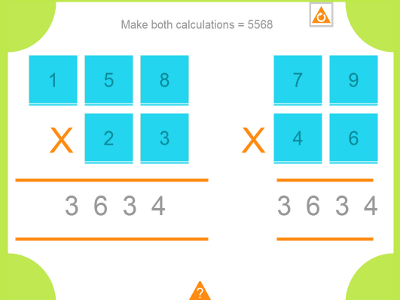A mathematical based puzzle for IWB, iPad and Android that requires some careful numeracy thinking and some informed trial and error.

When the resource opens you will see that both multiplication calculations give the same result of 3634. The highest number that can be the answer for both calculations using each of the nine digits once is 5568. The challenge is to arrange the numbers until both calculations equal 5568.  The resource will take care of the calculating but the player will have to think hard about the results of multiplication to make progress.  Random moving of tiles is likely to take a long time!

Tap one tile and then another to swap them.

Go to math puzzle.